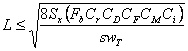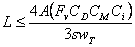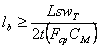Online Span Calculator Help
##### Span Calculations

Lumber design values used to calculate maximum horizontal spans include modulus of elasticity (E), bending strength (Fb), and shear strength (Fv).  Bearing strength in compression perpendicular to grain (Fcp) is used to determine the minimum required bearing length at each end of joists and rafters.  Calculated spans incorporate design value adjustments appropriate for repetitive-member use (Cr = 1.15), duration of load (CD), lumber size (CF), wet service conditions (CM), and incised lumber (Ci).  The 2012 National Design Specification® for Wood Construction (NDS®) specifies appropriate magnitudes for lumber design values and adjustment factors.

Maximum horizontal joist and rafter spans are taken as the smallest span (L) calculated from the following three formulas:based on bending strength (Fb)

where   s = spacing between joists or rafters

Sx = section modulus for strong-axis bending of joist or rafter

wT = total distributed load (D + L, or D + Lr, or D + S)
supported by joist or rafter, in terms of load per unit areabased on deflection limit and modulus of elasticity (E)

where   Ix = strong axis moment of inertia for joist or rafter

wL = distributed live load (L or Lr) or distributed snow
load (S) supported by joist or rafter,
in terms of load per unit area

deflection constant = constant term in denominator of
deflection limit (e.g., L/360)based on shear strength (Fv)

where   A = cross-sectional area of joist or rafter

##### Bearing Length

The minimum required bearing length (lb) at each end of a joist or rafter is determined from the following formula:where   t = thickness of joist or rafter